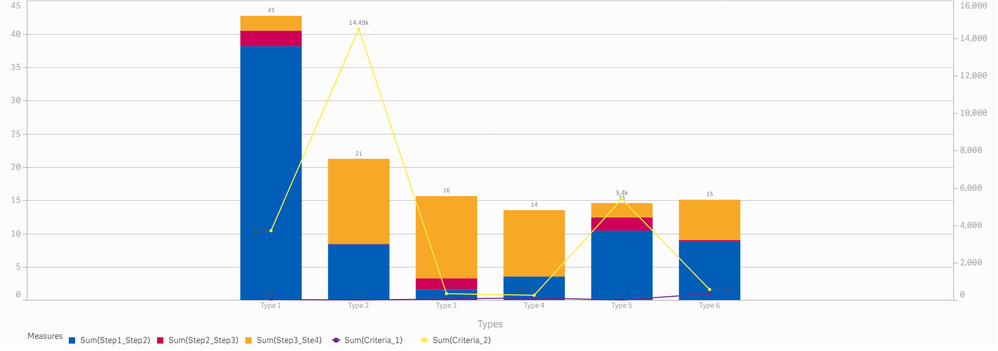Announcements
Applications are open for the 2024 Qlik Luminary Program. Apply by December 15 here.
cancel
Showing results for
Did you mean:Contributor III

## How to handle the very large scale view across left and right Y axis in the Qlik Sense

Hello All,

Can you please help in explaining the better way to put the graph with a view when we have drastic ranges over multiple y-axis measures

Data

 Types Sum(Step1_Step2) Sum(Step2_Step3) Sum(Step3_Ste4) Sum(Criteria_1) Sum(Criteria_2) Type 1 38.1 2.3 2.2 52 3,714.6 Type 2 8.4 0.1 12.8 15 14,495.0 Type 3 1.5 1.7 12.4 69 353.3 Type 4 3.5 0.0 10.0 115 261.9 Type 5 10.4 2.0 2.1 11 5,400.9 Type 6 8.9 0.1 6.1 342 564.0

Using this data we wanted to show that Sum(Step1_Step2) Sum(Step2_Step3) Sum(Step3_Ste4) as Stacked bar information and Sum(Criteria_1) as one Y-axis line and Sum(Criteria_2)  as another Y-axis line and problem being the

1. Sum(Criteria_1) is way smaller compared to Sum(Criteria_2)
2. Sum of Sum(Step1_Step2) Sum(Step2_Step3) Sum(Step3_Ste4) is way smaller than Sum(Criteria_1) sometimes

Hence we are not able to fit the Sum(Criteria_1) which is rarely visible proper in the graph and I have attached the graph in the section below, Can you please help me provide a better solution and some kind of hacks where we can solve this problemLabels (2)

• ### General Question

3 RepliesEmployee

Hi Qlikuser3 - I sent this to a few of my colleagues to see what might be the best way to approach this.

Regards,

Mike T

Qlik

Regards,
Mike Tarallo
QlikContributor III
AuthorEmployee

Imho, having measures of several orders of magnitude apart is probably not best solved by putting everything in to one chart.

I favor like in this article (https://outlier.ai/data-driven-daily/data-visualization-comparing-multiple-measures-on-the-same-plot...) to reduce to two measures and a dual axis. Another option could be to normalize (if possible) the measures to %.

Thanks,

PatricTags
Community Browser